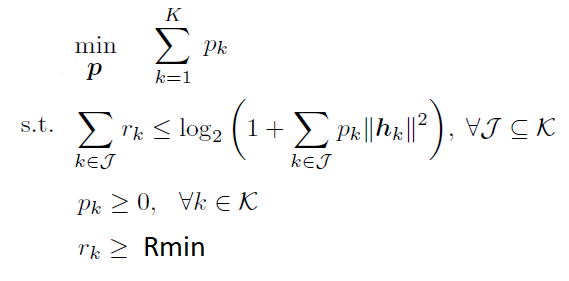# Cannot perform the operation: {real affine} ./ {real affine}

I am trying to simulate the following convex problem:Basically, trying to minimize the power of two users in UL transmission which are assigned to the same subcarrier of a BS. Therefore, interference is also included for the calculation of the rate.

However, only the interference of the weak user h_w < h_s is included, assuming the strong user can apply a Successive interference cancellation (SIC) technique.

I have formulated the following code, but I must have done something wrong because I get the following error message: Cannot perform the operation: {real affine} ./ {real affine}
Can I get some feedback please, so I can figure out what I am doing wrong?`Preformatted text`

``````K= 2;
R_min = 10^-2;
Power_total = 10;
h = complex(randn(2,1),randn(2,1))/sqrt(2); %CSI

cvx_begin
variable p(2) nonnegative
minimize sum(p)
subject to
expression Interference(1,2);
for i=1:K
Interference(i) = 0;
for j=1:K
if (i ~= j) && (abs(h(i)) > abs(h(j)))
Interference(i) = Interference(i) + p(j)*norm(h(j))^2;
end
end
end

% Minimum rate
log(1+p(1)*norm(h(1))^2/(1+Interference(1))) >= R_min
log(1+p(2)*norm(h(2))^2/(1+Interference(2))) >= R_min

% MAC contraints
log(1+p(1)*norm(h(1))^2/(1+Interference(1))) <= log(1+p(1)*norm(h(1))^2)
log(1+p(2)*norm(h(2))^2/(1+Interference(2))) <= log(1+p(2)*norm(h(2))^2)
log(1+p(1)*norm(h(1))^2/(1+Interference(1)))+log(1+p(2)*norm(h(2))^2/(1+Interference(2))) <= log(1+p(1)*norm(h(1))^2 + p(2)*norm(h(2))^2)

sum(p) <= Power_total
p >= 0
cvx_end``````

The error is occurring in the constraint
`log(1+p(1)*norm(h(1))^2/(1+Interference(1))) >= R_min`
because `Interference(1)` is (equal to) `p(1)`
This constraint is convex because the LHS is conccave in p(1).

You ought to be able to use the approach, modified to handle the `norm(h(1))^2` multiplying p(1). in @Michal_Adamaszek’s answer in Can CVX solve this kind of function {x-log(1+x/(x+1))} which will be DCP-compliant, and hence accepted by CVX.

The constraint
`log(1+p(2)*norm(h(2))^2/(1+Interference(2))) >= R_min`
is o.k. as is because Interference(2) = 0.

However, the MAC constraints violate the DCP rules, and will be rejected by CVX. Why isn't CVX accepting my model? READ THIS FIRST! . The 2nd of these MAC constraint trivially holds, and so can be eliminated.

However, the first MAC constraint is non-convex; if the constraint is formulated as `LHS - RHS <= 0`, then `LHS -RHS` has 2nd derivative with respect to p(1) being either positive or negative for various values of p(1) >= 0; therefore it is non-convex I haven’t checked the convexity of the 3rd of the MAC constraints.

1 Like

You are right, the MAC constraints violate the DCP rules, and will be rejected by CVX.

About @Michal_Adamaszek 's answer in Can CVX solve this kind of function {x-log(1+x/(x+1))} , it’s not the same case because Interference(1) = p(2)*norm(h(2))^2. Therefore, in my case becomes:

log(1+x/(1+y))

which cannot be solved with the way @Michal_Adamaszek has proposed

I wrote

modified to handle the `norm(h(1))^2` multiplying p(1).

Just use `norm(h(1))^2` in place of `0.01` in @Michal_Adamaszek’s answer.
I.e.,

``````multiplier = norm(h(1))^2;;
log(1 + multiplier - multiplier*inv_pos(p(1)+1)) >= R_min
``````

This solution works for any value of `multiplier` which is nionnegative.

Ok, got it. Thank you.

How can i write the following constraints, so that cvx would accept it?

`````` r(1) >=  log( 1+p(1)*norm(h(1))^2 / (1 + p(2)*norm(h(2))^2))

r(2) >=  log( 1+p(2)*norm(h(2))^2 / (1 + p(1)*norm(h(1))^2))``````

Those constraints are not convex. The right-hand sides are indefinite, i.e., neither convex nor concave. The numerators inside the log are “going the wrong way”.